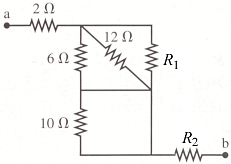# Problem: What is the equivalent resistance between points a and b in the figure below, in which R1 = 4 and R2 = 8 ?

###### FREE Expert Solution

In this problem, we are combining resistors in parallel/series. We'll need the following equations:

Equivalent resistance for resistors in parallel:

89% (415 ratings)###### Problem Details

What is the equivalent resistance between points a and b in the figure below, in which R1 = 4 and R2 = 8 ?